# Class 12 Maths NCERT Solutions for Chapter 4 Determinants Exercise 4.4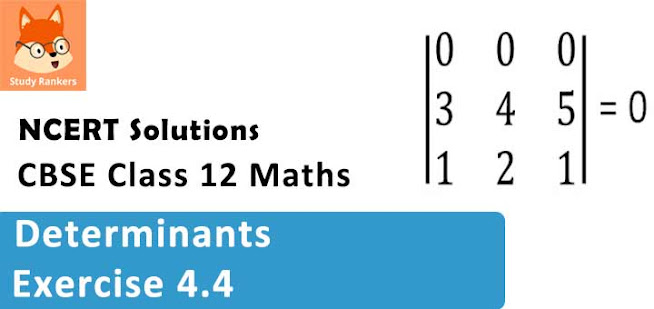### Determinants Exercise 4.4 Solutions

1. Write Minors and Cofactors of the elements of following determinants :
(i)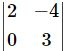(ii)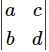Solution

(i) The given determinant isMinor of aij is Mij .
M11 = minor of element a11 = 3
M12 = minor of element a12 = 0
M21 = minor of element a21 = -4
M22 = minor of element a22 = 2
Cofactor of aij is Aij = (-1)i+j  Mij .
∴ A11 = (-1)1+1 M11 = (-1)2 (3) = 3
A12 = (-1)1+2 M12 = (-1)3 (0) = 0
A21 = (-1)2+1 M21 = (-1)3 (-4) = 4
A22 = (-1)2+2 M22 = (-1)4 (2) = 2

(ii) The given determinant isMinor of element aij is Mij .
∴ M11 = minor of element a11 = d
M12 = minor of element a12 = b
M21 = minor of element a21 = c
M22 = minor of element a22 = a
Cofactor of aij is Aij = (-1)i+j  Mij .
∴ A11 = (-1)1+1 M11 = (-1)2 (d) = d
A12 = (-1)1+2 M12 = (-1)3 (b) = -b
A21 = (-1)2+1 M21 = (-1)3 (c) = -c
A22 = (-1)2+2 M22 = (-1)4 (a) = a

2. Write Minors and Cofactors of the elements of following determinants:
(i)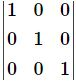(ii)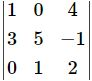Solution

(i) The given determinant isBy the definition of minors and cofactors, we have :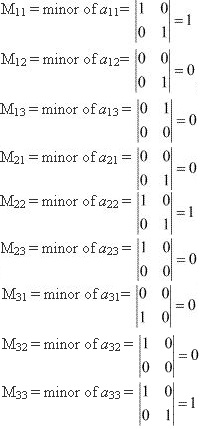A11 = cofactor of a11 = (-1)1+1 M11 = 1
A12 = cofactor of a12 = (-1)1+2 M12 = 0
A13 = cofactor of a13 = (-1)1+3 M13 = 0
A21 = cofactor of a21 = (-1)2+1 M21 = 0
A22 = cofactor of a22 = (-1)2+2 M22 = 1
A23 = cofactor of a23 = (-1)2+3 M23 = 0
A31 = cofactor of a31 = (-1)3+1 M31 = 0
A32 = cofactor of a32 = (-1)3+2 M32 = 0
A33 = cofactor of a33 = (-1)3+3 M33 = 1

(ii) The given determinant is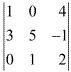By definition of minors and cofactors, we have :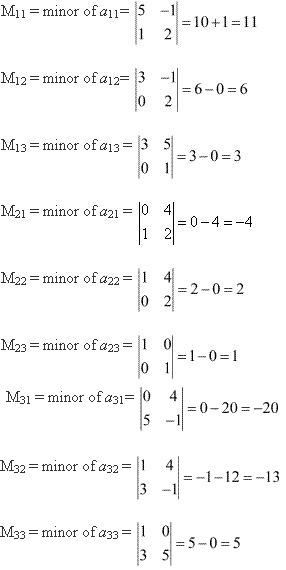A11 = cofactor of a11 = (-1)1+1 M11 = 11
A12 = cofactor of a12 = (-1)1+2 M12 = -6
A13 = cofactor of a13 = (-1)1+3 M13 = 3
A21 = cofactor of a21 = (-1)2+1 M21 = 4
A22 = cofactor of a22 = (-1)2+2 M22 = 2
A23 = cofactor of a23 = (-1)2+3 M23 = -1
A31 = cofactor of a31 = (-1)3+1 M31 = -20
A32 = cofactor of a32 = (-1)3+2 M32 = 13
A33 = cofactor of a33 = (-1)3+3 M33 = 5

3. Using Cofactors of elements of second row, evaluate ∆ =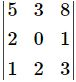Solution

He given determinant isWe have :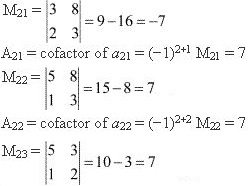A23 = cofactor of a23 = (-1)2+3 M23 = -7
We know that ∆ is equal to the sum of the product of the elements of the second row with their corresponding cofactors.
Δ = a21 A21 + a22 A22 + a23 A23 = 2(7) + 0(7) + 1(-7) = 14 - 7 = 7

4. sing Cofactors of elements of third column, evaluate Δ =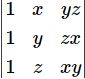Solution

The given determinant isWe have :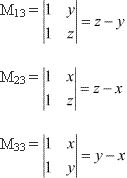A13 = cofactor of a13 = (-1)1+3 M13 = (z- y)
A23 = cofactor of a23 = (-1)2+3 M23 = -(z - x) = (x - z)
A33 = cofactor of a33 = (-1)3+3 M33 = (y - x)
We know that Δ is equal to the sum of the product of the elements of the second row with their corresponding cofactors.
∴ Δ = a21 A21 + a22 A22 + a23 A23
= yz(z - y) + zx(x - z) + xy(y - x)
= yz2 - y2 z  +x2 z - xz2 + xy2 - x2 y
= (x2 z - y2 z) + (yz2 - xz2 ) + (xy2 - x2 y)
= z(x2  - y2 ) + z2 (y -x) + xy(y -x)
= z(x - y)(x + y) + z2 (y -x) + xy(y - x)
= (x - y)[zx - zy - z2 - xy]
= (x - y) [z(x -z) + y(z - x)]
= (x - y) (z - x)[-z + y]
= (x - y)(y - z)(z - x)
Hence, Δ = (x - y)(y - z)(z - x) .

5. If ∆ =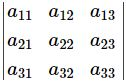and Aij  is Cofactors of aij , then value of Δ is given by
(A) a11 A31+ a12 A32 + a13 A33
(B) a11 A11+ a12 A21 + a13 A31
(C) a21 A11+ a22 A12 + a23 A13
(D) a11 A11+ a21 A21 + a31 A31

Solution

We know that :

∆ = Sum of the product of the elements of a column (or a row) with their corresponding cofactors
∆ = a21 A21 + a22 A22 + a23 A23
Hence, the value of Δ is given by the expression given in alternative D.